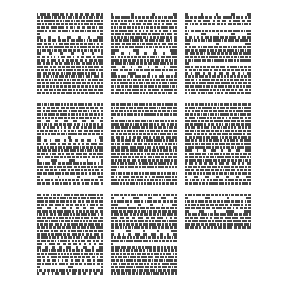ggpage features

Variable paragraph length

The ggpage package allows for variable length of paragraphs if the text doesn’t hold information regarding paragraphs. This is done by supplying the variable.paragraph.length argument in ggpage_build with a function that generate random natural numbers. Such as the rpois function.

library(magrittr)
library(dplyr)
library(ggpage)

book <- tinderbox %>%
tidytext::unnest_tokens(text, text)
#> Warning: replacing previous import 'dplyr::vars' by 'rlang::vars' when

ggpage_build(book, para.fun = rpois, lambda = 75) %>%
ggpage_plot()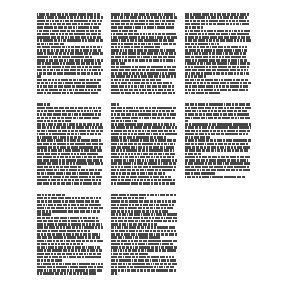ggpage_build(book, para.fun = rpois, lambda = 75) %>%
ggpage_plot()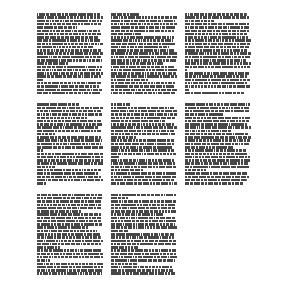ggpage_build(book, para.fun = rpois, lambda = 500) %>%
ggpage_plot()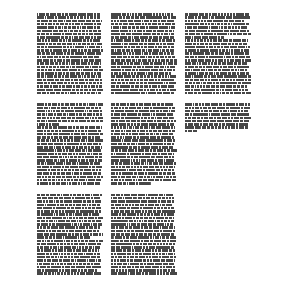ggpage_build(book, para.fun = rpois, lambda = 500) %>%
ggpage_plot()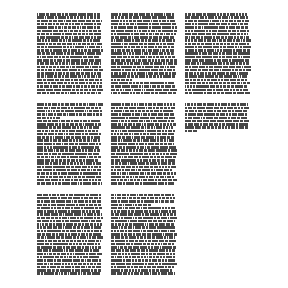Show paper

With the newest update ggpage allows you to draw a rectangle around each “page”.

ggpage_build(tinderbox) %>%
ggpage_plot(paper.show = TRUE)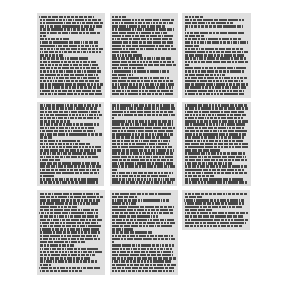It allows you use specify a color both as a constant,

ggpage_build(tinderbox) %>%
ggpage_plot(paper.show = TRUE, paper.color = "pink")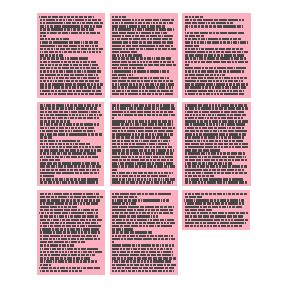or by a string of colors. In this case a faded rainbow.

ggpage_build(tinderbox) %>%
ggpage_plot(paper.show = TRUE, paper.color = rainbow(9), paper.alpha = 0.3)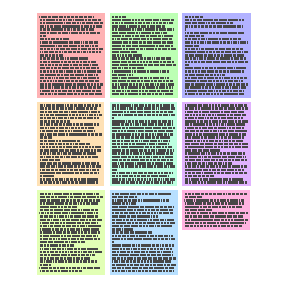Show page number

It can be hard to see the order of the pages in the standard vizualization. However it is possible to add a page number in the sides or corners of each page.

directions <- c("top", "top-right", "right", "bottom-right", "bottom",
"bottom-left", "left", "top-left")
ggpage_build(tinderbox) %>%
ggpage_plot(page.number = directions)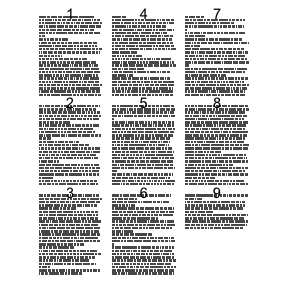ggpage_build(tinderbox) %>%
ggpage_plot(page.number = directions)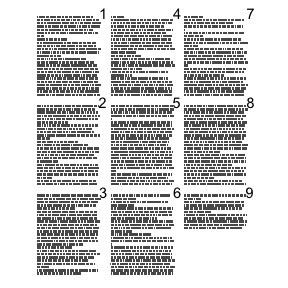ggpage_build(tinderbox) %>%
ggpage_plot(page.number = directions)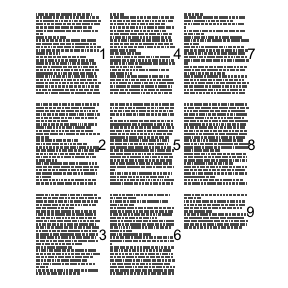ggpage_build(tinderbox) %>%
ggpage_plot(page.number = directions)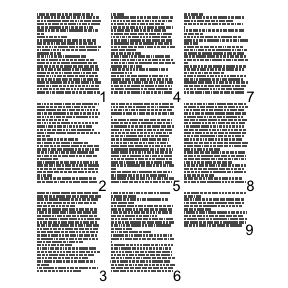ggpage_build(tinderbox) %>%
ggpage_plot(page.number = directions)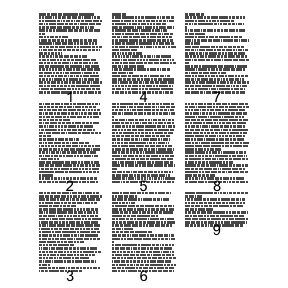ggpage_build(tinderbox) %>%
ggpage_plot(page.number = directions)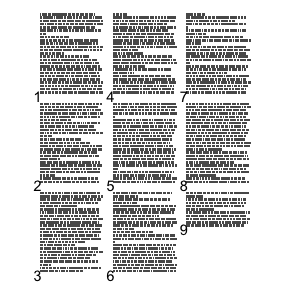ggpage_build(tinderbox) %>%
ggpage_plot(page.number = directions)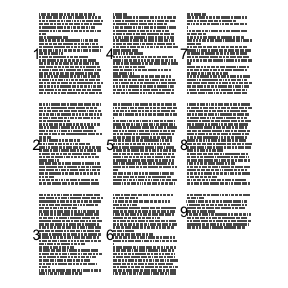ggpage_build(tinderbox) %>%
ggpage_plot(page.number = directions)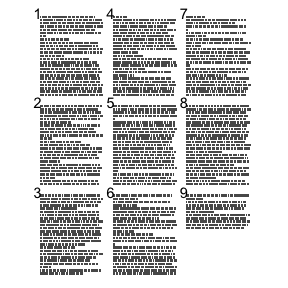Variable page length

The package also support a variable page length. This can be helpful when a collection of smaller texts are considered in the same vizualisation such as tweets.

tinderbox %>%
mutate(short = ceiling(seq_len(nrow(tinderbox)) / 10)) %>%
ggpage_build(page.col = "short", lpp = 10) %>%
ggpage_plot()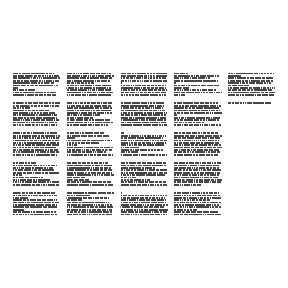Centering

ggpage now supports left, right and both-sided alignments.

ggpage_build(tinderbox, align = "right") %>%
ggpage_plot()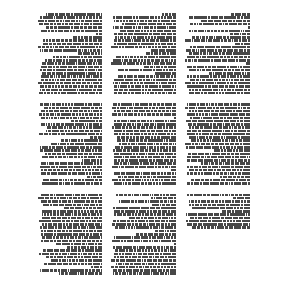ggpage_build(tinderbox, align = "both") %>%
ggpage_plot()